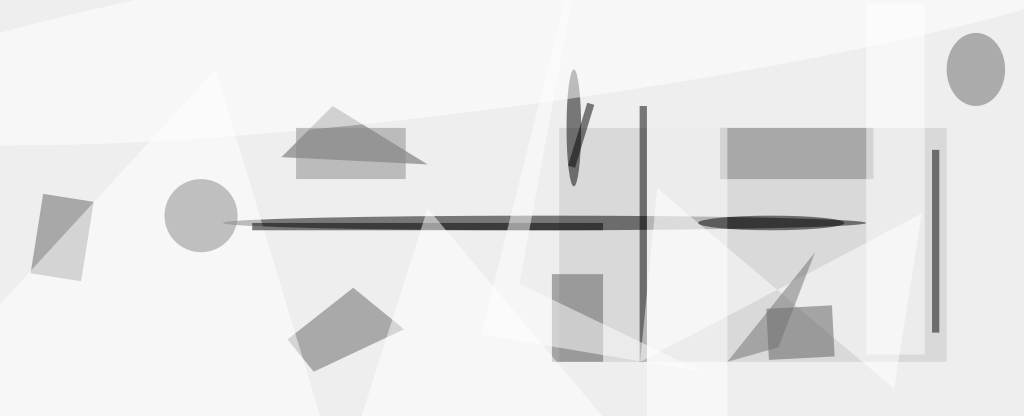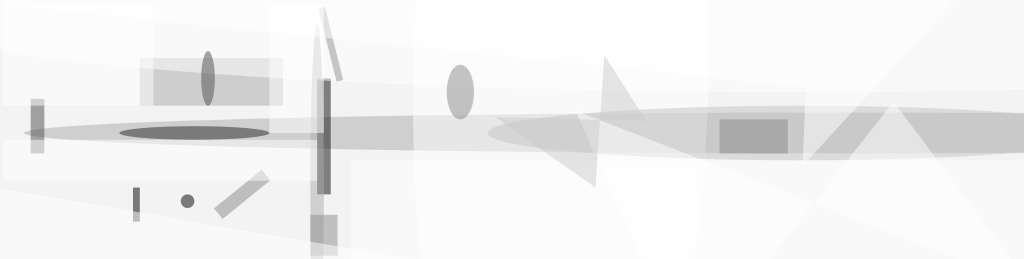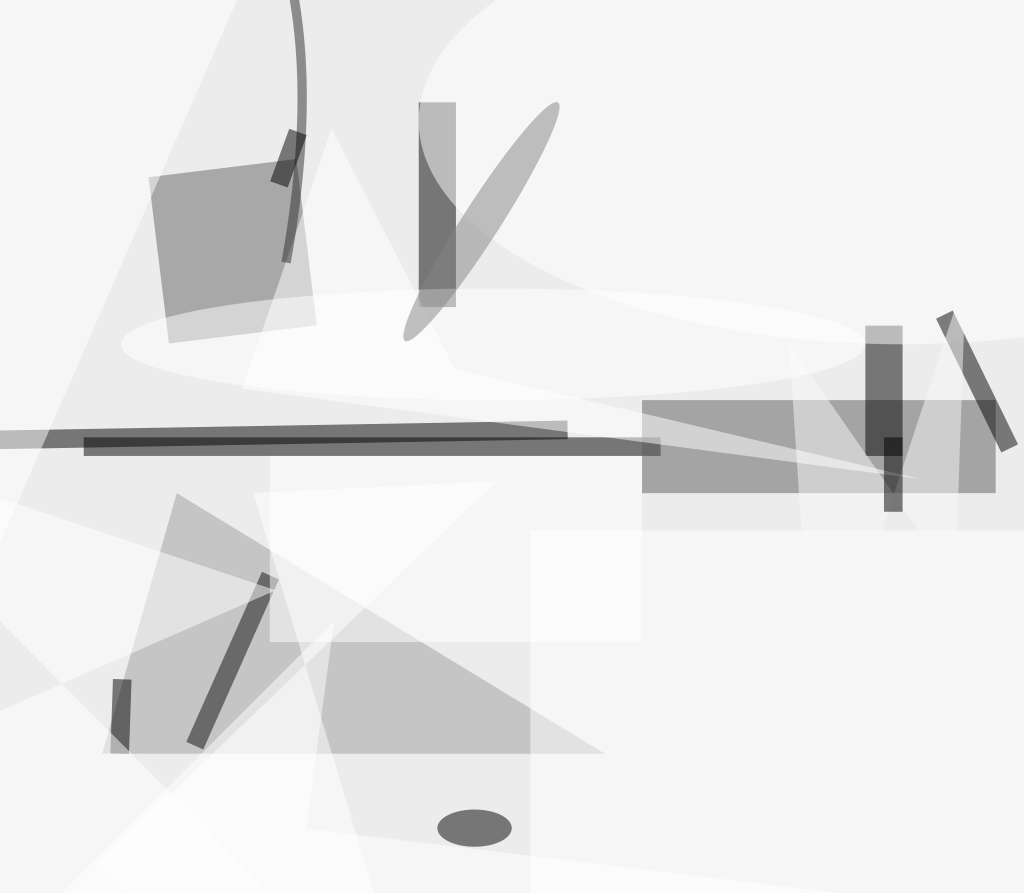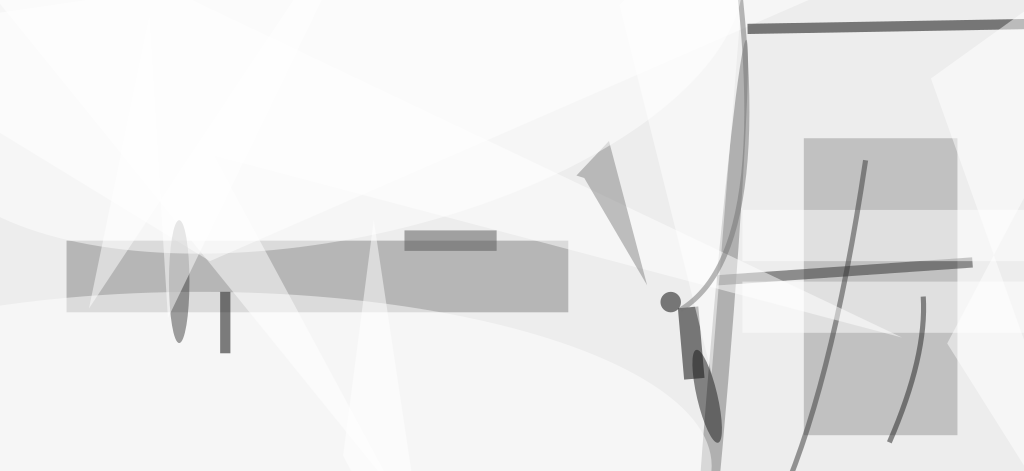Equations have been derived to fill a gap in previously published standard collections of stress-and-strain formulas: These are equations for deflection of a thin circular membrane that is clamped rigidly around its periphery and subjected to differential pressure. Related equations were described in "Deflection and Stress in Preloaded Square Membrane," (GSC-13367, Vol. 15, No. 9 (September 1991), page 96 and "Deflection and Stress in Preloaded Rectangular Membrane," (GSC-13561), Vol. 18, No. 3 (March 1994), page 100.

As in the cases of the square and rectangular membranes, the derivation of the equations for the circular membrane follows a strain-energy/virtual deflection approach, which is common in stress-and-strain problems of this kind. The displacements of the membrane under load are initially assumed to be of the form

1.and

2. u = r ( a - r)( c1 + c2 r)

where r is the radial coordinate, a is the radius of the clamping edge, w is the transverse displacement (that is, the deflection perpendicular to the nominal membrane plane) at radius r, w0 is the maximum transverse displacement, u is the radial displacement at radius r, and c1 and c2 are constants.

The radial and transverse strains are given, respectively, by

1.and

2.The strain energy associated with stretching of the membrane is given by

1.where E is Young's modulus, h is the thickness of the membrane, and ν is Poisson's ratio.

To calculate the deflection of the membrane, one must solve the foregoing equations to find c1, c2, and w0. First, one substitutes the right sides of equations (1) through (4) for the corresponding terms in equation (5). Using the resulting form of equation (5), one finds c1 and c2 by imposing the requirements that

1.and

2.Next, one imposes the requirement that the change in work done by the differential pressure acting through a virtual displacement equals the change in strain energy associated with the virtual displacement. If the virtual displacement is chosen to be δw δw0, then this requirement is expressed by the equation

1.where q is the differential pressure on the membrane. The solution for the maximum displacement is

1.where

2.This work was done by Alfonso Hermida of Goddard Space Flight Center. GSC-13783.##### NASA Tech Briefs Magazine

This article first appeared in the May, 1998 issue of NASA Tech Briefs Magazine.

Read more articles from the archives here.